Home » A Hands-on Tutorial to Learn Attention Mechanism For Image Caption Generation in Python

# A Hands-on Tutorial to Learn Attention Mechanism For Image Caption Generation in Python

## Overview

• Understand the attention mechanism for image caption generation
• Implement attention mechanism to generate caption in python

## Introduction

The attention mechanism is a complex cognitive ability that human beings possess. When people receive information, they can consciously ignore some of the main information while ignoring other secondary information.

This ability of self-selection is called attention. The attention mechanism allows the neural network to have the ability to focus on its subset of inputs to select specific features.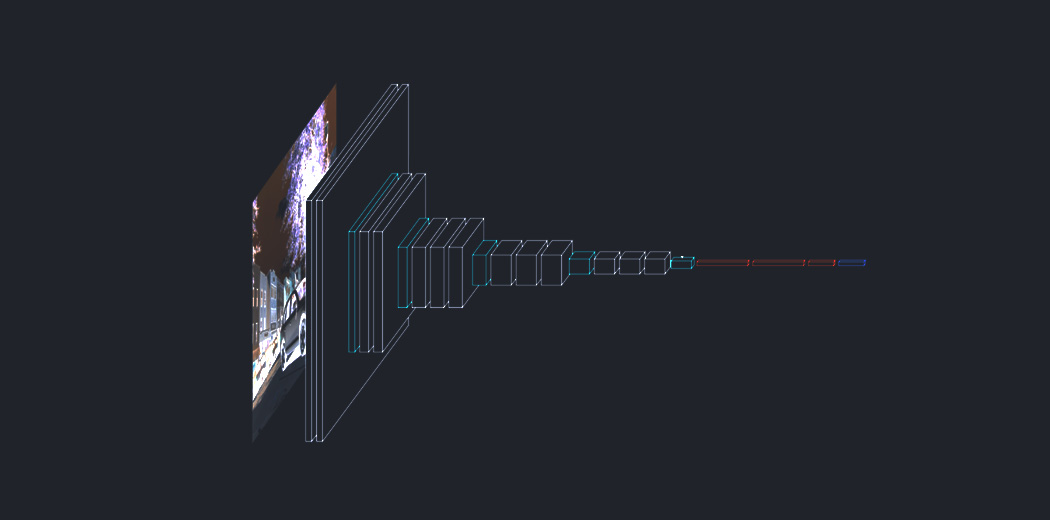In recent years, neural networks have fueled dramatic advances in image captioning. Researchers are looking for more challenging applications for computer vision and Sequence to Sequence modeling systems. They seek to describe the world in human terms. In the last article we had seen Image Captioning through a Merge architecture, today we’ll be looking at a much more complex yet refined design to tackle this problem.

Attention mechanism has been a go-to methodology for practitioners in the Deep Learning community. It was originally designed in the context of Neural Machine Translation using Seq2Seq Models, but today we’ll take a look at its implementation in Image Captioning.

Rather than compressing an entire image into a static representation, the Attention mechanism allows for salient features to dynamically come to the forefront as and when needed. This is especially important when there is a lot of clutter in an image.

Let’s take an example to understand better: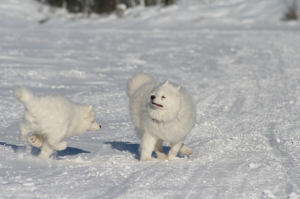Our aim would be to generate a caption like “two white dogs are running on the snow”. To accomplish this we will see how to implement a specific type of Attention mechanism called Bahdanau’s Attention or Local Attention.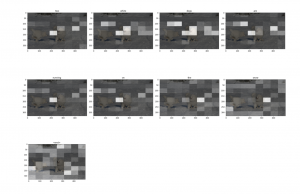In this way, we can see what parts of the image the model focuses on as it generates a caption. This implementation will require a strong background in deep learning.

### Prerequisites before you begin:

Let’s begin and gain a much deeper understanding of the concepts at hand!

1. Approach to the problem statement
2. Understanding the Dataset
3. Implementation
1. Importing required libraries
3. Model definition
4. Model training
5. Greedy Search and BLEU Evaluation
4. What’s Next?
5. End Notes

## Approach to the problem statement

The encoder-decoder image captioning system would encode the image, using a pre-trained Convolutional Neural Network that would produce a hidden state. Then, it would decode this hidden state by using an LSTM and generate a caption.

For each sequence element, outputs from previous elements are used as inputs, in combination with new sequence data. This gives the RNN networks a sort of memory which might make captions more informative and contextaware.

But RNNs tend to be computationally expensive to train and evaluate, so in practice, memory is limited to just a few elements. Attention models can help address this problem by selecting the most relevant elements from an input image.

With an Attention mechanism, the image is first divided into n parts, and we compute an image representation of each When the RNN is generating a new word, the attention mechanism is focusing on the relevant part of the image, so the decoder only uses specific parts of the image.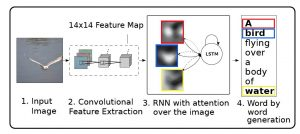In Bahdanau or Local attention, attention is placed only on a few source positions. As Global attention focuses on all source side words for all target words, it is computationally very expensive. To overcome this deficiency local attention chooses to focus only on a small subset of the hidden states of the encoder per target word.

Local attention first finds an alignment position and then calculates the attention weight in the left and right windows where its position is located and finally weights the context vector. The main advantage of local attention is to reduce the cost of the attention mechanism calculation.

In the calculation, the local attention is not to consider all the words on the source language side, but to predict the position of the source language end to be aligned at the current decoding according to a prediction function and then navigate through the context window, considering only the words within the window.

## Design of Bahdanau Attention

All hidden states of the encoder and the decoder are used to generate the context vector. The attention mechanism aligns the input and output sequences, with an alignment score parameterized by a feed-forward network. It helps to pay attention to the most relevant information in the source sequence. The model predicts a target word based on the context vectors associated with the source position and the previously generated target words.

To evaluate our captions in reference to the original caption we make use of an evaluation method called BLEU. It is the most widely used evaluation indicator. It is used to analyze the correlation of n-gram between the translation statement to be evaluated and the reference translation statement.

In this article, multiple images are equivalent to multiple source language sentences in the translation. The advantage of BLEU is that the granularity it considers is an n-gram rather than a word, considering longer matching information. The disadvantage of BLEU is that no matter what kind of n-gram is matched, it will be treated the same.

I hope this gives you an idea of how we are approaching this problem statement. Let’s dive into the implementation!

## Understanding the Dataset

I have used the Flickr8k dataset in which each image is associated with five different captions that describe the entities and events depicted in the image that were collected.

Flickr8k is a good starting dataset as it is small in size and can be trained easily on low-end laptops/desktops using a CPU.

Our dataset structure is as follows:-

• Flick8k/
• Flick8k_Dataset/ :- contains the 8000 images
• Flick8k_Text/
• Flickr8k.token.txt:- contains the image id along with the 5 captions

I defined an 80:20 split to create training and test data.

## Let’s Implement Attention Mechanism for Caption Generation!

#### Step 1:- Import the required libraries

Here we will be making use of Tensorflow for creating our model and training it. The majority of the code credit goes to TensorFlow tutorials. You can make use of Google Colab or Kaggle notebooks if you want a GPU to train it.

```import string
import numpy as np
import pandas as pd
from numpy import array

from PIL import Image
import pickle
from collections import Counter
import matplotlib.pyplot as plt

import sys, time, os, warnings
warnings.filterwarnings("ignore")
import re

import keras
import tensorflow as tf
from tqdm import tqdm
from nltk.translate.bleu_score import sentence_bleu

from keras.utils import to_categorical
from keras.utils import plot_model
from keras.models import Model
from keras.layers import Input
from keras.layers import Dense, BatchNormalization
from keras.layers import LSTM
from keras.layers import Embedding
from keras.layers import Dropout
from keras.callbacks import ModelCheckpoint
from keras.preprocessing.text import Tokenizer
from keras.applications.vgg16 import VGG16, preprocess_input

from sklearn.utils import shuffle
from sklearn.model_selection import train_test_split
from sklearn.utils import shuffle```

Define our image and caption path and check how many total images are present in the dataset.

```image_path = "/content/gdrive/My Drive/FLICKR8K/Flicker8k_Dataset"
dir_Flickr_text = "/content/gdrive/My Drive/FLICKR8K/Flickr8k_text/Flickr8k.token.txt"
jpgs = os.listdir(image_path)

print("Total Images in Dataset = {}".format(len(jpgs)))```

Output: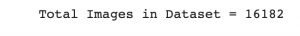We create a dataframe to store the image id and captions for ease of use.

```file = open(dir_Flickr_text,'r')
file.close()

datatxt = []
for line in text.split('\n'):
col = line.split('\t')
if len(col) == 1:
continue
w = col.split("#")
datatxt.append(w + [col.lower()])

data = pd.DataFrame(datatxt,columns=["filename","index","caption"])
data = data.reindex(columns =['index','filename','caption'])
data = data[data.filename != '2258277193_586949ec62.jpg.1']
uni_filenames = np.unique(data.filename.values)

Output: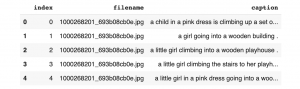Next, let’s visualize a few images and their 5 captions:

```npic = 5
npix = 224
target_size = (npix,npix,3)
count = 1

fig = plt.figure(figsize=(10,20))
for jpgfnm in uni_filenames[10:14]:
filename = image_path + '/' + jpgfnm
captions = list(data["caption"].loc[data["filename"]==jpgfnm].values)
count += 1

plt.axis('off')
ax.plot()
ax.set_xlim(0,1)
ax.set_ylim(0,len(captions))
for i, caption in enumerate(captions):
ax.text(0,i,caption,fontsize=20)
count += 1
plt.show()```

Output: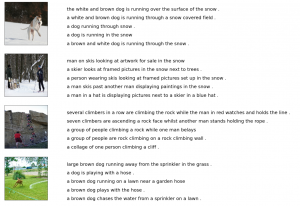Next let’s see what our current vocabulary size is:-

```vocabulary = []
for txt in data.caption.values:
vocabulary.extend(txt.split())
print('Vocabulary Size: %d' % len(set(vocabulary)))```

Output: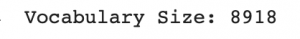Next perform some text cleaning such as removing punctuation, single characters, and numeric values:

```def remove_punctuation(text_original):
text_no_punctuation = text_original.translate(string.punctuation)
return(text_no_punctuation)

def remove_single_character(text):
text_len_more_than1 = ""
for word in text.split():
if len(word) > 1:
text_len_more_than1 += " " + word
return(text_len_more_than1)

def remove_numeric(text):
text_no_numeric = ""
for word in text.split():
isalpha = word.isalpha()
if isalpha:
text_no_numeric += " " + word
return(text_no_numeric)

def text_clean(text_original):
text = remove_punctuation(text_original)
text = remove_single_character(text)
text = remove_numeric(text)
return(text)

for i, caption in enumerate(data.caption.values):
newcaption = text_clean(caption)
data["caption"].iloc[i] = newcaption```

Now let’s see the size of our vocabulary after cleaning-

```clean_vocabulary = []
for txt in data.caption.values:
clean_vocabulary.extend(txt.split())
print('Clean Vocabulary Size: %d' % len(set(clean_vocabulary)))```

Output: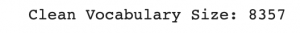Next, we save all the captions and image paths in two lists so that we can load the images at once using the path set. We also add ‘< start >’ and ‘< end >’ tags to every caption so that the model understands the starting and end of each caption.

```PATH = "/content/gdrive/My Drive/FLICKR8K/Flicker8k_Dataset/"
all_captions = []
for caption  in data["caption"].astype(str):
caption = '<start> ' + caption+ ' <end>'
all_captions.append(caption)

all_captions[:10]```

Output: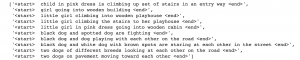```all_img_name_vector = []
for annot in data["filename"]:
full_image_path = PATH + annot
all_img_name_vector.append(full_image_path)

all_img_name_vector[:10]```

Output: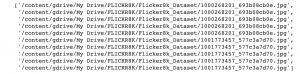Now you can see we have 40455 image paths and captions.

```print(f"len(all_img_name_vector) : {len(all_img_name_vector)}")
print(f"len(all_captions) : {len(all_captions)}")```

Output: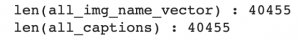We will take only 40000 of each so that we can select batch size properly i.e. 625 batches if batch size= 64. To do this we define a function to limit the dataset to 40000 images and captions.

```def data_limiter(num,total_captions,all_img_name_vector):
train_captions, img_name_vector = shuffle(total_captions,all_img_name_vector,random_state=1)
train_captions = train_captions[:num]
img_name_vector = img_name_vector[:num]
return train_captions,img_name_vector

train_captions,img_name_vector = data_limiter(40000,total_captions,all_img_name_vector)```

#### Step 3:- Model Definition

Let’s define the image feature extraction model using VGG16. We must remember that we do not need to classify the images here, we only need to extract an image vector for our images. Hence we remove the softmax layer from the model. We must all preprocess all the images to the same size, i.e, 224×224 before feeding them into the model.

```def load_image(image_path):
img = tf.image.decode_jpeg(img, channels=3)
img = tf.image.resize(img, (224, 224))
img = preprocess_input(img)
return img, image_path

image_model = tf.keras.applications.VGG16(include_top=False, weights='imagenet')
new_input = image_model.input
hidden_layer = image_model.layers[-1].output
image_features_extract_model = tf.keras.Model(new_input, hidden_layer)

image_features_extract_model.summary()```

Output: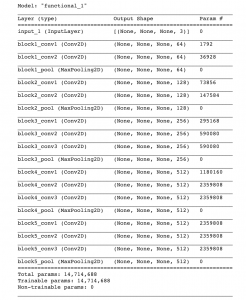Next, let’s Map each image name to the function to load the image:-

```encode_train = sorted(set(img_name_vector))
image_dataset = tf.data.Dataset.from_tensor_slices(encode_train)

We extract the features and store them in the respective .npy files and then pass those features through the encoder.NPY files store all the information required to reconstruct an array on any computer, which includes dtype and shape information.

```%%time
for img, path in tqdm(image_dataset):
batch_features = image_features_extract_model(img)
batch_features = tf.reshape(batch_features,
(batch_features.shape, -1, batch_features.shape))

for bf, p in zip(batch_features, path):
path_of_feature = p.numpy().decode("utf-8")
np.save(path_of_feature, bf.numpy())```

Next, we tokenize the captions and build a vocabulary of all the unique words in the data. We will also limit the vocabulary size to the top 5000 words to save memory. We will replace words not in vocabulary with the token < unk >

```top_k = 5000
tokenizer = tf.keras.preprocessing.text.Tokenizer(num_words=top_k,
oov_token="<unk>",
filters='!"#\$%&()*+.,-/:;[email protected][\]^_`{|}~ ')

tokenizer.fit_on_texts(train_captions)
train_seqs = tokenizer.texts_to_sequences(train_captions)

train_seqs = tokenizer.texts_to_sequences(train_captions)

Let’s visualize the padded training and captions and the tokenized vectors:

`train_captions[:3]`

Output: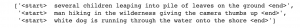`train_seqs[:3]`

Output: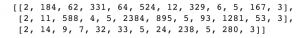Next, we can calculate the max and min length of all captions:

```def calc_max_length(tensor):
return max(len(t) for t in tensor)
max_length = calc_max_length(train_seqs)

def calc_min_length(tensor):
return min(len(t) for t in tensor)
min_length = calc_min_length(train_seqs)

print('Max Length of any caption : Min Length of any caption = '+ str(max_length) +" : "+str(min_length))```

Output: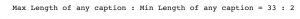Next, Create training and validation sets using an 80-20 split:

`img_name_train, img_name_val, cap_train, cap_val = train_test_split(img_name_vector,cap_vector, test_size=0.2, random_state=0)`

Define the parameters for training:

```BATCH_SIZE = 64
BUFFER_SIZE = 1000
embedding_dim = 256
units = 512
vocab_size = len(tokenizer.word_index) + 1
num_steps = len(img_name_train) // BATCH_SIZE
features_shape = 512
attention_features_shape = 49```

Next, let’s create a tf.data dataset to use for training our model.

```def map_func(img_name, cap):
return img_tensor, cap
dataset = tf.data.Dataset.from_tensor_slices((img_name_train, cap_train))

# Use map to load the numpy files in parallel
dataset = dataset.map(lambda item1, item2: tf.numpy_function(
map_func, [item1, item2], [tf.float32, tf.int32]),
num_parallel_calls=tf.data.experimental.AUTOTUNE)

dataset = dataset.shuffle(BUFFER_SIZE).batch(BATCH_SIZE)
dataset = dataset.prefetch(buffer_size=tf.data.experimental.AUTOTUNE)```

Next, let’s define the encoder-decoder architecture with attention. The architecture defined in this article is similar to the one described in the paperShow and Tell: A Neural Image Caption Generator”:-

The VGG-16 Encoder is defined below:-

```class VGG16_Encoder(tf.keras.Model):
# This encoder passes the features through a Fully connected layer
def __init__(self, embedding_dim):
super(VGG16_Encoder, self).__init__()
# shape after fc == (batch_size, 49, embedding_dim)
self.fc = tf.keras.layers.Dense(embedding_dim)
self.dropout = tf.keras.layers.Dropout(0.5, noise_shape=None, seed=None)

def call(self, x):
#x= self.dropout(x)
x = self.fc(x)
x = tf.nn.relu(x)
return x   ```

We define our RNN based on GPU/CPU capabilities-

```def rnn_type(units):
if tf.test.is_gpu_available():
return tf.compat.v1.keras.layers.CuDNNLSTM(units,
return_sequences=True,
return_state=True,
recurrent_initializer='glorot_uniform')
else:
return tf.keras.layers.GRU(units,
return_sequences=True,
return_state=True,
recurrent_activation='sigmoid',
recurrent_initializer='glorot_uniform')```

Next, define the RNN Decoder with Bahdanau Attention:

```'''The encoder output(i.e. 'features'), hidden state(initialized to 0)(i.e. 'hidden') and
the decoder input (which is the start token)(i.e. 'x') is passed to the decoder.'''

class Rnn_Local_Decoder(tf.keras.Model):
def __init__(self, embedding_dim, units, vocab_size):
super(Rnn_Local_Decoder, self).__init__()
self.units = units
self.embedding = tf.keras.layers.Embedding(vocab_size, embedding_dim)
self.gru = tf.keras.layers.GRU(self.units,
return_sequences=True,
return_state=True,
recurrent_initializer='glorot_uniform')

self.fc1 = tf.keras.layers.Dense(self.units)

self.dropout = tf.keras.layers.Dropout(0.5, noise_shape=None, seed=None)
self.batchnormalization = tf.keras.layers.BatchNormalization(axis=-1, momentum=0.99, epsilon=0.001, center=True, scale=True, beta_initializer='zeros', gamma_initializer='ones', moving_mean_initializer='zeros', moving_variance_initializer='ones', beta_regularizer=None, gamma_regularizer=None, beta_constraint=None, gamma_constraint=None)

self.fc2 = tf.keras.layers.Dense(vocab_size)

# Implementing Attention Mechanism
self.Uattn = tf.keras.layers.Dense(units)
self.Wattn = tf.keras.layers.Dense(units)
self.Vattn = tf.keras.layers.Dense(1)

def call(self, x, features, hidden):
# features shape ==> (64,49,256) ==> Output from ENCODER
# hidden shape == (batch_size, hidden_size) ==>(64,512)
# hidden_with_time_axis shape == (batch_size, 1, hidden_size) ==> (64,1,512)

hidden_with_time_axis = tf.expand_dims(hidden, 1)

# score shape == (64, 49, 1)
# Attention Function
'''e(ij) = f(s(t-1),h(j))'''
''' e(ij) = Vattn(T)*tanh(Uattn * h(j) + Wattn * s(t))'''

score = self.Vattn(tf.nn.tanh(self.Uattn(features) + self.Wattn(hidden_with_time_axis)))

# self.Uattn(features) : (64,49,512)
# self.Wattn(hidden_with_time_axis) : (64,1,512)
# tf.nn.tanh(self.Uattn(features) + self.Wattn(hidden_with_time_axis)) : (64,49,512)
# self.Vattn(tf.nn.tanh(self.Uattn(features) + self.Wattn(hidden_with_time_axis))) : (64,49,1) ==> score

# you get 1 at the last axis because you are applying score to self.Vattn
# Then find Probability using Softmax
'''attention_weights(alpha(ij)) = softmax(e(ij))'''

attention_weights = tf.nn.softmax(score, axis=1)

# attention_weights shape == (64, 49, 1)
# Give weights to the different pixels in the image
''' C(t) = Summation(j=1 to T) (attention_weights * VGG-16 features) '''

context_vector = attention_weights * features
context_vector = tf.reduce_sum(context_vector, axis=1)

# Context Vector(64,256) = AttentionWeights(64,49,1) * features(64,49,256)
# context_vector shape after sum == (64, 256)
# x shape after passing through embedding == (64, 1, 256)

x = self.embedding(x)
# x shape after concatenation == (64, 1,  512)

x = tf.concat([tf.expand_dims(context_vector, 1), x], axis=-1)
# passing the concatenated vector to the GRU

output, state = self.gru(x)
# shape == (batch_size, max_length, hidden_size)

x = self.fc1(output)
# x shape == (batch_size * max_length, hidden_size)

x = tf.reshape(x, (-1, x.shape))

# Adding Dropout and BatchNorm Layers
x= self.dropout(x)
x= self.batchnormalization(x)

# output shape == (64 * 512)
x = self.fc2(x)

# shape : (64 * 8329(vocab))
return x, state, attention_weights

def reset_state(self, batch_size):
return tf.zeros((batch_size, self.units))

encoder = VGG16_Encoder(embedding_dim)
decoder = Rnn_Local_Decoder(embedding_dim, units, vocab_size)```

Next, we define the loss function and optimizers:-

```optimizer = tf.keras.optimizers.Adam()
loss_object = tf.keras.losses.SparseCategoricalCrossentropy(
from_logits=True, reduction='none')

def loss_function(real, pred):
loss_ = loss_object(real, pred)

return tf.reduce_mean(loss_)```

#### Step 4:- Model Training

Next, let’s define the training step. We make use of a technique called Teacher Forcing, which is the technique where the target word is passed as the next input to the decoder. This technique helps to learn the correct sequence or correct statistical properties for the sequence, quickly.

```loss_plot = []

@tf.function
def train_step(img_tensor, target):
loss = 0
# initializing the hidden state for each batch
# because the captions are not related from image to image

hidden = decoder.reset_state(batch_size=target.shape)
dec_input = tf.expand_dims([tokenizer.word_index['<start>']] * BATCH_SIZE, 1)

features = encoder(img_tensor)
for i in range(1, target.shape):
# passing the features through the decoder
predictions, hidden, _ = decoder(dec_input, features, hidden)
loss += loss_function(target[:, i], predictions)

# using teacher forcing
dec_input = tf.expand_dims(target[:, i], 1)

total_loss = (loss / int(target.shape))
trainable_variables = encoder.trainable_variables + decoder.trainable_variables

return loss, total_loss```

Next, we train the model:-

```EPOCHS = 20
for epoch in range(start_epoch, EPOCHS):
start = time.time()
total_loss = 0

for (batch, (img_tensor, target)) in enumerate(dataset):
batch_loss, t_loss = train_step(img_tensor, target)
total_loss += t_loss

if batch % 100 == 0:
print ('Epoch {} Batch {} Loss {:.4f}'.format(
epoch + 1, batch, batch_loss.numpy() / int(target.shape)))
# storing the epoch end loss value to plot later
loss_plot.append(total_loss / num_steps)

print ('Epoch {} Loss {:.6f}'.format(epoch + 1,
total_loss/num_steps))

print ('Time taken for 1 epoch {} sec\n'.format(time.time() - start))```

Let’s plot the error graph:

```plt.plot(loss_plot)
plt.xlabel('Epochs')
plt.ylabel('Loss')
plt.title('Loss Plot')
plt.show()```

Output: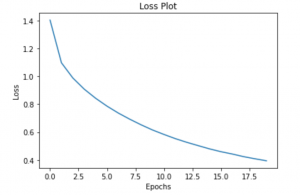Step 5:- Greedy Search and BLEU Evaluation

Let’s define our greedy method of defining captions:

```def evaluate(image):
attention_plot = np.zeros((max_length, attention_features_shape))

hidden = decoder.reset_state(batch_size=1)
img_tensor_val = image_features_extract_model(temp_input)
img_tensor_val = tf.reshape(img_tensor_val, (img_tensor_val.shape, -1, img_tensor_val.shape)

features = encoder(img_tensor_val)
dec_input = tf.expand_dims([tokenizer.word_index['<start>']], 0)
result = []

for i in range(max_length):
predictions, hidden, attention_weights = decoder(dec_input, features, hidden)
attention_plot[i] = tf.reshape(attention_weights, (-1, )).numpy()
predicted_id = tf.argmax(predictions).numpy()
result.append(tokenizer.index_word[predicted_id])

if tokenizer.index_word[predicted_id] == '<end>':
return result, attention_plot

dec_input = tf.expand_dims([predicted_id], 0)
attention_plot = attention_plot[:len(result), :]

return result, attention_plot```

Also, we define a function to plot the attention maps for each word generated as we saw in the introduction-

```def plot_attention(image, result, attention_plot):
temp_image = np.array(Image.open(image))
fig = plt.figure(figsize=(10, 10))
len_result = len(result)
for l in range(len_result):
temp_att = np.resize(attention_plot[l], (8, 8))
ax.set_title(result[l])
img = ax.imshow(temp_image)
ax.imshow(temp_att, cmap='gray', alpha=0.6, extent=img.get_extent())

plt.tight_layout()
plt.show()```

Finally, let’s generate a caption for the image at the start of the article and see what the attention mechanism focuses on and generates-

```# captions on the validation set
rid = np.random.randint(0, len(img_name_val))
image = '/content/gdrive/My Drive/FLICKR8K/Flicker8k_Dataset/2319175397_3e586cfaf8.jpg'

# real_caption = ' '.join([tokenizer.index_word[i] for i in cap_val[rid] if i not in ])
result, attention_plot = evaluate(image)

# remove <start> and <end> from the real_caption
first = real_caption.split(' ', 1)
real_caption = 'Two white dogs are playing in the snow'

#remove "<unk>" in result
for i in result:
if i=="<unk>":
result.remove(i)

for i in real_caption:
if i=="<unk>":
real_caption.remove(i)

#remove <end> from result
result_join = ' '.join(result)
result_final = result_join.rsplit(' ', 1)

real_appn = []
real_appn.append(real_caption.split())
reference = real_appn
candidate = result

score = sentence_bleu(reference, candidate)
print(f"BELU score: {score*100}")

print ('Real Caption:', real_caption)
print ('Prediction Caption:', result_final)
plot_attention(image, result, attention_plot)```

Output: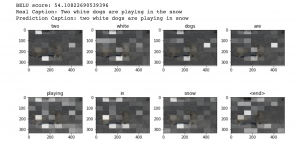You can see we were able to generate the same caption as the real caption. Let’s try it out for some other images from the test set.

```rid = np.random.randint(0, len(img_name_val))
image = img_name_val[rid]
start = time.time()
real_caption = ' '.join([tokenizer.index_word[i] for i in cap_val[rid] if i not in ])
result, attention_plot = evaluate(image)

first = real_caption.split(' ', 1)
real_caption = first.rsplit(' ', 1)

#remove "<unk>" in result
for i in result:
if i=="<unk>":
result.remove(i)

#remove <end> from result
result_join = ' '.join(result)
result_final = result_join.rsplit(' ', 1)

real_appn = []
real_appn.append(real_caption.split())
reference = real_appn
candidate = result_final

print ('Real Caption:', real_caption)
print ('Prediction Caption:', result_final)

plot_attention(image, result, attention_plot)
print(f"time took to Predict: {round(time.time()-start)} sec")

Image.open(img_name_val[rid])```

Output: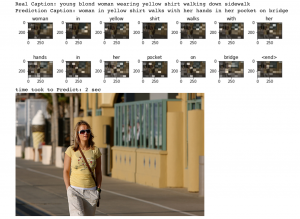You can see even though our caption is quite different from the real caption, it is still very accurate. It was able to identify the yellow shirt of the woman and her hands in the pocket.

Let’s see another one:

```rid = np.random.randint(0, len(img_name_val))
image = img_name_val[rid]

real_caption = ' '.join([tokenizer.index_word[i] for i in cap_val[rid] if i not in ])
result, attention_plot = evaluate(image)

# remove <start> and <end> from the real_caption
first = real_caption.split(' ', 1)
real_caption = first.rsplit(' ', 1)

#remove "<unk>" in result
for i in result:
if i=="<unk>":
result.remove(i)

for i in real_caption:
if i=="<unk>":
real_caption.remove(i)

#remove <end> from result
result_join = ' '.join(result)
result_final = result_join.rsplit(' ', 1)

real_appn = []
real_appn.append(real_caption.split())
reference = real_appn
candidate = result

score = sentence_bleu(reference, candidate)
print(f"BELU score: {score*100}")

print ('Real Caption:', real_caption)
print ('Prediction Caption:', result_final)

plot_attention(image, result, attention_plot)```

Output: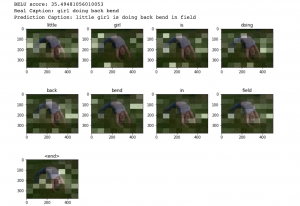Here we can see our caption defines the image better than one of the real captions.

And there it is!  We have successfully implemented the Attention Mechanism for generating Image Captions.

## What’s Next?

The attention mechanism is highly utilized in recent years and is just the start to much more state of the art systems. Things you can implement to improve your model:-

• Make use of the larger datasets, especially the MS COCO dataset or the Stock3M dataset which is 26 times larger than MS COCO.
• Implement different attention mechanisms like Adaptive Attention with Visual Sentinel and. Semantic Attention
• Implementing a Transformer based model which should perform much better than an LSTM.
• Implementing better architecture for image feature extraction like Inception, Xception, and Efficient networks.

## End Notes

This was quite an interesting look at the Attention mechanism and how it applies to deep learning applications. There has been immense research in the attention mechanism and achieving a state of the art results.

Make sure to try some of my suggestions to improve the performance of our generator and share your results with me!

You can also read this article on our Mobile APP